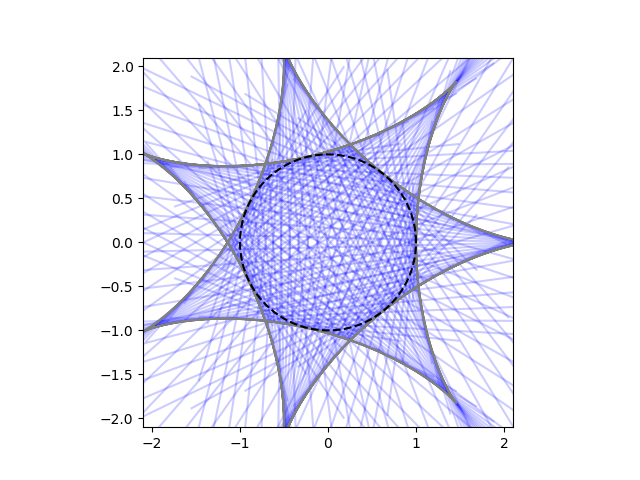# Hypocycloids

In the previous post, I showed how to plot “envelopes of epicycloids.” This post will consider a variation on the same theme, hypocycloids.For the epicycloid post, we imagined two ants crawling around a circle at different speeds, and drawing lines between their positions at various times. Although the ants were traveling at different speeds, they were both moving in the same orientation.

For hypocycloids, we imagine our two ants again, but this time they’re traveling in opposite directions, one clockwise and one counterclockwise.

The position of the two ants at time t are

(cos pt, sin pt)

and

(cos qt, sin qt)

as before, and again p and q are integers, but this time q is negative and

p > |q| > 0  > q.

Also, we need to make one more change: the tangent lines have to be longer. If we just draw lines that begin and end on the circle, we’ll get a dense mesh of lines but not see the hypocycloid. The hypocycloid is on the outside of the unit circle, not inside. A plot further down will make this clear.

The image at the top of the post corresponds to p = 5 and q = -2. Here are a couple more examples.

First, p = 4 and q = -3.Next, p = 7 and q = -3.The dark area in the middle is what you’d see if you only connected points on the circle rather than extending the lines. More on that below.

The equation of the hypocycloid itself, the figure carved out by the tangent lines, isHere’s a close-up of the figure at the top of the post with more detail: axes, the hypocycloid drawn in gray, and the unit circle drawn with a dashed black line.The Python code to produce the graphs in this post is similar to the code in the previous post.

One thing to note: it’s important that the alpha level is set to a small value. The blue lines in this post use alpha = 0.2. This makes the lines translucent, and so places where many lines overlap are darker than isolated lines. With the default value of alpha = 1, all lines would be opaque and it would be harder to see what’s going on.